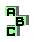MEDIANS

 r22 first Brocard triangle, symmedian lines d centroid d first Brocard parabola d symmedian lines r221 Johnson triangle, orthocenter r439 centroid, Steiner circumellipse r557 symmedian lines, Simson line, circumcircle r559 anticomplement, perspective, Kiepert hyperbola, Steiner point r629 perspective, complement, centroid, circumcenter, symmedian point r845 circumcircle, symmedian lines, orthic axis r851 orthiac triangles, point X(185) r853 sinorthiac triangles, symmedian point c33 r1201 Steiner circumellipse r1332 symmedian lines, Brocard points c75 altitudes r1679 centroid, orthocenter, cevian circle r1680 centroid, orthocenter, cevian circle, point X(858) r1681 centroid, orthocenter, cevian circle, point X(468), altitude feet r2030 circumcenter, centroid, ninecenter r2042 centroid, concyclic points